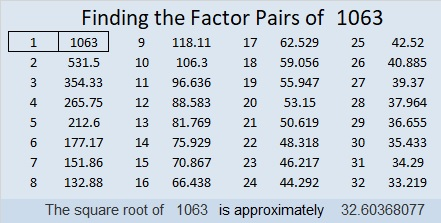# 1063 and Level 1

Lucky you found this puzzle today! You can solve it by writing the factors 1 to 12 in both the first column and the top row so that the given clues are the products of the corresponding factors.Print the puzzles or type the solution in this excel file: 12 factors 1063-1072

Now I’ll share a little information about the number 1063:

• 1063 is a prime number. 1061 and 1063 are twin primes.
• Prime factorization: 1063 is prime.
• The exponent of prime number 1063 is 1. Adding 1 to that exponent we get (1 + 1) = 2. Therefore 1063 has exactly 2 factors.
• Factors of 1063: 1, 1063
• Factor pairs: 1063 = 1 × 1063
• 1063 has no square factors that allow its square root to be simplified. √1063 ≈ 32.60368

How do we know that 1063 is a prime number? If 1063 were not a prime number, then it would be divisible by at least one prime number less than or equal to √1063 ≈ 32.6. Since 1063 cannot be divided evenly by 2, 3, 5, 7, 11, 13, 17, 19, 23, 29 or 31, we know that 1063 is a prime number.1063 is the sum of seven consecutive prime numbers:
137 + 139 + 149 + 151 + 157 + 163 + 167 = 1063

1063 is a high flying palindrome in one other base:
It’s 747 in BASE 12 because 7(12²) + 4(12) + 7(1) = 1063

# What Kind of Prime Is 859?

A prime number is a positive number that has exactly two factors, one and itself. (One has only one factor, so it is not a prime number.)

• 859 is the 149th prime number.

A twin prime is a set of two prime numbers in which the second prime number is two more that the first prime number.

• 859 is the second prime number in the 34th twin prime: (857, 859).

A prime triplet is a set of three consecutive prime numbers in which the last number is six more than the first number. Prime triplets always contain a set of twin primes.

• 859 is in the 27th and 28th prime triplets: (853, 857, 859) and (857, 859, 863).

A prime quadruplet is a set of four consecutive prime numbers in which the last number is eight more than the first number. Prime quadruplets always contain TWO sets of overlapping prime triplets.

• Even though prime numbers (853, 857, 859, 863) contain two sets of overlapping prime triplets, they do NOT form a prime quadruplet because the last number is ten more than the first number. Other than (5, 7, 11, 13), all prime quadruplets are prime decades whose last digits are 1, 3, 7, and 9, in THAT order.

There are other prime constellations like prime quintuplets and prime sextuplets, but each of those has to contain a prime quadruplet in it, so 859 isn’t in any of those.

859÷4 = 214 R3. Since that wasn’t R1, we know that 859 is NOT the hypotenuse of ANY Pythagorean triples.

Now you know what kind of prime 859 is.

Here’s today’s puzzle:Print the puzzles or type the solution on this excel file: 10-factors-853-863

• 859 is a prime number.
• Prime factorization: 859 is prime.
• The exponent of prime number 859 is 1. Adding 1 to that exponent we get (1 + 1) = 2. Therefore 859 has exactly 2 factors.
• Factors of 859: 1, 859
• Factor pairs: 859 = 1 × 859
• 859 has no square factors that allow its square root to be simplified. √859 ≈ 29.3087How do we know that 859 is a prime number? If 859 were not a prime number, then it would be divisible by at least one prime number less than or equal to √859 ≈ 29.3. Since 859 cannot be divided evenly by 2, 3, 5, 7, 11, 13, 17, 19, 23, or 29, we know that 859 is a prime number.